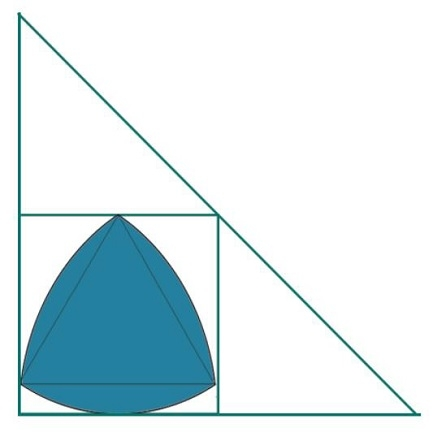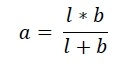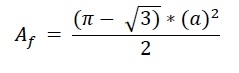# Biggest Reuleaux Triangle within a Square which is inscribed within a Right angle Triangle?

Here we will see the area of biggest Reuleaux triangle inscribed within a square, that square is inscribed inside one right angled triangle. The side of the square is ‘a’. The height of the Reuleaux triangle is x. The base of the triangle is b, height of the triangle is l, and the hypotenuse is h.We know that the side of square inscribed in a Right angled triangle with height l and base b is −The height of the Reuleaux triangle is same as a. So a = x. So the area of Reuleaux triangle is −## Example

#include <iostream>
#include <cmath>
using namespace std;
float areaReuleaux(float l, float b) { //l and b are height and base of right angled triangle
if (l < 0 || b < 0) //either l or b is negative it is invalid
return -1;
float a = (l*b)/(l+b);
float area = ((3.1415 - sqrt(3)) * (a) * (a))/2;
return area;
}
int main() {
float l = 5;
float b = 12;
cout << "Area of Reuleaux Triangle: " << areaReuleaux(l, b);
}

## Output

Area of Reuleaux Triangle: 8.77858